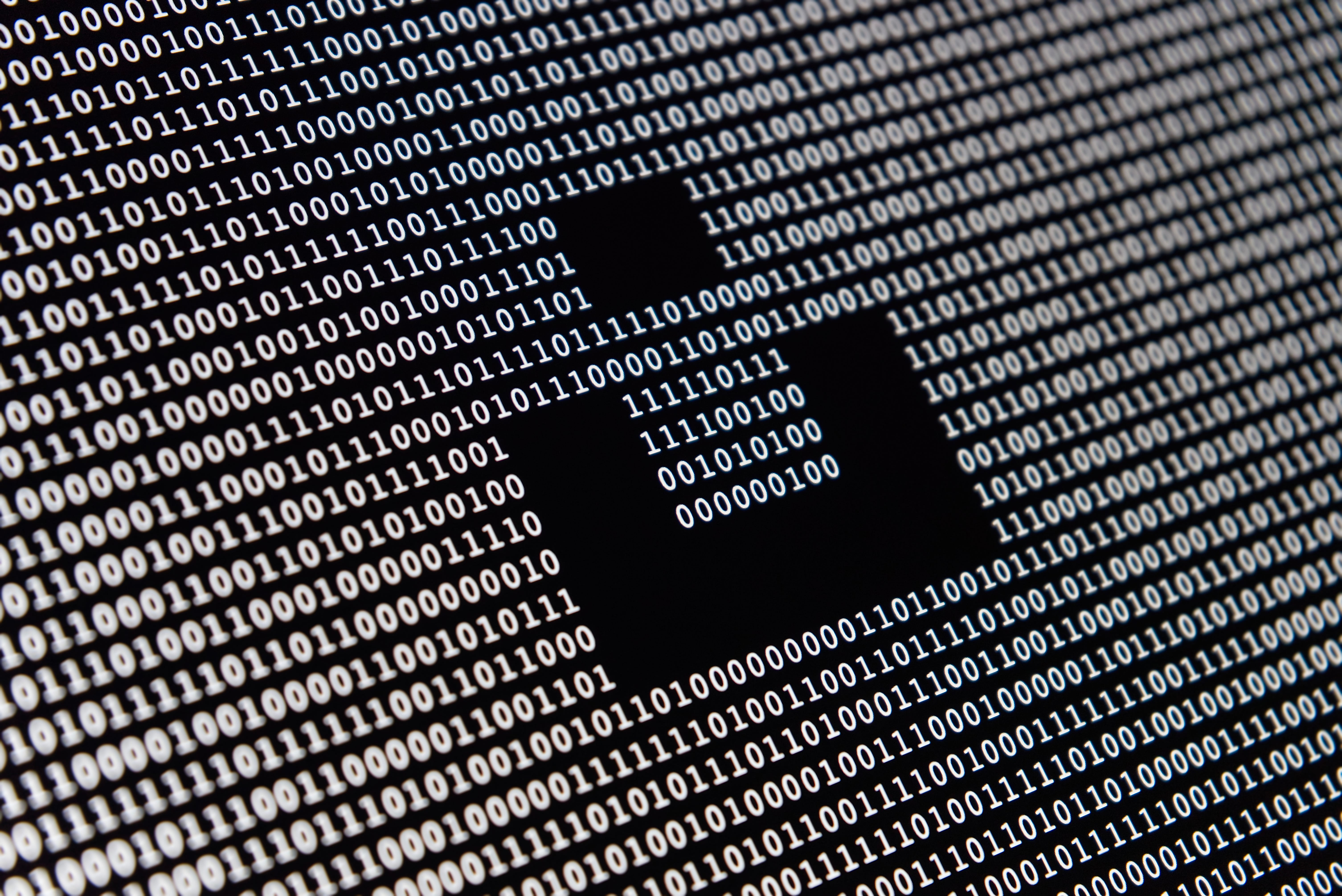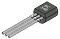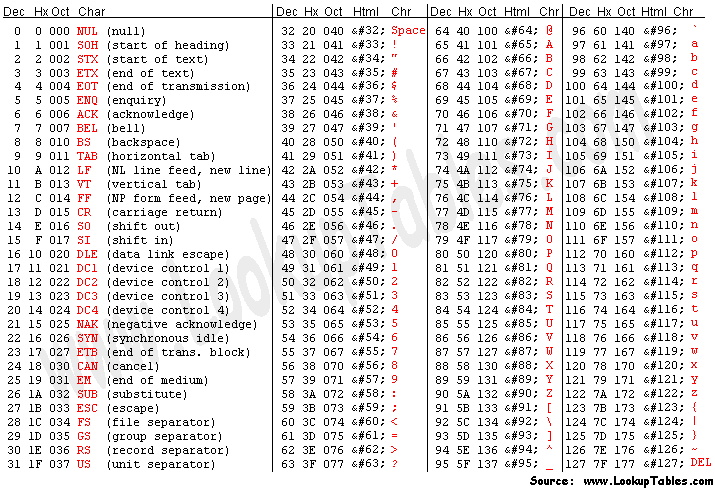# Binary: What You Need to Know

There are 10 types of people in this world, those who understand binary and those who don’t. — UnknownPhoto by Alexander Sinn on Unsplash

# Why do we use the decimal system?

The history of counting methods is rich and complicated, so unfortunately, much of it has to be passed over in this article. The main point is that humans eventually started to count in the decimal system, aka “base-10”, because we have 10 fingers (“dec” means 10 in Latin and Greek). Nowadays, counting this way may seem like second nature, however, the number 10 has no significant meaning in the universe. It just seems important to us because we chose our counting system to have 10 characters: 0, 1, 2, 3, 4, 5, 6, 7, 8, and 9. We could have easily chosen any other number.

Some ancient civilizations did use other numbers, such as the Egyptians counting some hand knuckles in a base-12 system, the Mayans counting on their hands and toes for a base-20 system, and the Babylonians counting in a base-60 system by counting on all their hand knuckles. Binary is simply just a base-2 system. These are all examples of positional notation systems, where the location of the digit signifies how “important it” is. This is an alternative to the sign-value notation that Roman Numerals use.

# What is binary?

When many people think of binary, they may remember the scene from the Matrix where an endless stream of 0’s and 1’s tearing across a screen — looking quite intimidating. However, binary is no more than just another way to count. The Greek and Latin word, “bi”, means 2. This is because there are 2 distinct values that a single number in binary can have: 0 and 1. Instead of calling a single value a “digit”, as we do in decimal, we call a single binary value a “bit”.

## Decimal

So how do we count in binary? It is no different than how we count in decimal. Consider the decimal number, 4039. This can be represented as:
4 1000’s, 0 100's, 3 10’s, and 9 1’s.

In mathematical terms:
4039 = 4x1000 + 0x100+ 3x10 + 9x1.

There is one more generalization we can make here. Notice that as the digits move to the left by one, the value that they get multiplied by increases by 10x ( 1 ->10 -> 100 -> 1000 -> …). So we can also write this as:
4039 = 4(10³) +0(10²) + 3(10¹) + 9(10⁰).

All positional notation systems operate in this same way. Decimal is a base-10 system, so that’s why the powers of 10 are used. Also, the digits can’t get any larger than 10–1 = 9. Differently based systems have different powers and different limits, but they all operate similarly.

## Binary

Now that we took a deep dive into how decimal numbers work, we can talk about binary numbers. Binary numbers operate just like digital numbers, except for 2 differences.

1. The values increase by powers of 2 as the bits move from right to left.
2. The maximum value that a bit can be is 2–1=1. So it can be either 0 or 1.

As an example, let’s convert the digital number 53 to binary. There are multiple techniques to do this, but the main idea is to find the correct combination of powers of 2’s that add up to the desired value. This is quite difficult to get the hang of, but a bonus section at the bottom of this article will explain how to do this with just pencil and paper. The solution is below:
53 = 1(2⁵) + 1(2⁴) + 0(2³) + 1(2²) + 0(2¹) + 1(2⁰)

To ensure that this is correct, this equation will be simplified and solved:
53 = 2⁵ + 2⁴ + 2² + 2⁰
53 = 32 +16 + 4 + 1
53 = 53

We can see that this equation correctly solves for the original number! But how do we convert this to binary? The first equation actually already has the answer. The coefficients in front of each of the powers of 2 make up the binary solution: 110101. To ensure that this isn’t confused for the decimal number, 110,101, the common notation to show that a number is binary is to lead it with a “0b”. So we would write our solution as 0b110101.

## Binary to decimal

It is much easier to understand how to go from binary to decimal than from decimal to binary. We will do this with the binary number, 0b1011.

The first step is to simply convert the binary number to its positional notation:
0b1011 = 1(2³) + 0(2²) + 1(2¹) + 1(2⁰)

Then, simplify the equation:
0b1011 = 2³ + 2¹ + 1
0b1011 = 8 + 2 + 1
0b1011 = 11
Now we have our final answer, 0b1011 in binary is equal to 11 in decimal!

# Why do we use binary?

## Transistors

Now that we understand what binary numbers are and how to convert to and from them… why should we care? Well, binary numbers are significantly easier to represent in computers than decimal numbers are. This is because of the transistor, which is an electrical device that forms the foundation of all computer systems.Simple Transistor [from Instructables]

Transistors can store either a “1”, which means that it has a voltage applied to it (Usually 3.3 or 5 Volts), or a “0”, which means that it has 0 Voltage applied to it. Engineers and scientists have been able to continually decrease the size of transistors since they were invented in 1947. You may even be familiar with Moore’s Law, which states that the number of transistors that can fit on a dense integrated circuit of the same size doubles roughly every 2 years. The point is, transistors are fundamental to all operations of a computer.

## Why not base-3 or higher?

Precision Issues
If one were to try and invent a computer that can operate on a base-3 system instead of a base-2 system, they would run into quite a few issues. The first issue would be that they would have to reinvent the transistor to have 3 distinct states. One possible solution would be that 0–1.67 Volts could be equal to “0”, then 1.67–3.33 Volts could be equal to a “1”, then 3.33–5 Volts could be equal to a “2”.

Although theoretically possible, this would increase the chances of getting errors because the true voltage levels fluctuate some. Instead of the 5V gaps that a binary system has (0V to 5V), this trinary system would only have 1.67V gaps between voltages. This would require a more precise system to distinguish between different states which would make it easier for errors to happen when the voltage fluctuates, which it tends to do. If one were to try and implement a base-4 or higher system as computer hardware, this issue would only get worse.

Propositional Logic
Additionally, binary systems have the incredible advantage of being able to use propositional logic, which is the branch of mathematics concerned with logical statements that can be either True or False. Many real-life scenarios can be represented with propositional logic, so it is much easier to program a computer with this binary logic. This field would make for another fascinating article that I may have to get to in the future.

# Arithmetic

## Addition

Arithmetic in binary is just like how it is in decimal. It is easy to work out by hand with pencil and paper. Let’s add the binary values 0b1001 and 0b0101. First, notice that we can add any number of leading zeros to binary numbers just as we can in decimal: 009 is the same as just 9.

Addition happens just as it does with decimal, where the numbers are added together element-wise right to left, and if a number gets too large, it carries a 1 to the next pair. In this example, the rightmost bits added together, 1+1 = 10, overflows the maximum value that a bit can have, so we carry over a 1 to the next pair and leave the rightmost result bit as a 0. Then, addition carries on as normal to the remaining pairs, where in this case, there is no more overflow.

## Subtraction

Subtraction can occur in a very similar way where each bit is subtracted from another and if a bit goes below 0, it borrows it from the following bit. This doesn’t require much explanation because it is quite boring and it isn’t actually used in practice. How computers actually perform subtraction on binary numbers is a by using a very cool trick to their advantage.

Previously, we have ignored the number of bits in a number and basically assumed that they are infinite. However, computers only set aside a certain amount of storage for each number that they store. For example, if a number is stored in 8 bits, the largest number that it can be is 0b11111111 = 255. If a number larger than 255 is attempted to be stored in an 8-bit storage space, the space “resets” and only stores the smallest 8 bits of that number. For example, 266 would be the 9 bit 0b100000001, but since there are only 8 bits, it would result in losing the leftmost bit and only being 0b00000001. This is called an “Overflow”. Usually, computer programs are written in such a way that this is hardly an issue, but it is important to know for a trick that makes subtraction much easier.

If we have two binary numbers, A and B, and we want to find the result of A minus B. This can also be found by adding A to what is called the “Two’s Complement” of B. The Two’s Complement of a binary number can be calculated with 2 steps.
1. Invert the bits. (0's become 1's and 1's become 0's)
2. Add one to the result.

For example. If we wanted to calculate 8 (0b1000) minus 6 (0b0110), we first find the Two’s Complement of 6. Let’s assume that it is stored in 4 bits:
Original: 0b0110
Inverted bits: 0b1001
Add 1: 0b1010
Result (Two’s Compliment of 6): 0b1010

Now we can add simply add this result to 8 to find out what 8–6 is:
0b1000 + 0b1010
= 0b10010
However, since the size of the data storage is only 4 bits, the data overflows and only the smallest 4 bits are kept. So, we are left with:
0b0010 = 2
Which is the correct answer for 8–6 = 2! This Two’s Complement subtraction trick makes it much easier to build hardware that can do arithmetic, which will be touched on in the next section.

## Multiplication and Division

One of the most fascinating parts of computers is that addition, subtraction, multiplication, and division can all be performed with simple(ish) circuits. Data is stored in transistors and operated on by going through arithmetic blocks at incredible rates of speed. This is a pretty advanced topic that will take quite a bit of time to explain, so an article explaining more about this will definitely be coming soon.

## How to represent characters

An introduction to binary wouldn’t be complete without mentioning how it is also used to represent characters. There is no natural conversion of characters to 0’s and 1’s, so all assignments must be chosen by hand. Unfortunately, there is not an assignment that everyone agreed on. Instead, there are several competing standards such as ASCII, Unicode, and many others from different countries. Luckily, this doesn’t cause too many issues because many programming languages check for and fix these errors. An example of an ASCII table, which provides a mapping of numbers to characters, is shown below.ASCII Table [from asciitable]

## Decimal to binary by hand (Bonus)

As promised, I will provide a method for converting from decimal to binary by hand. This is not a critical topic for understanding binary, but it may be interesting to some, so here it is. The basic idea is to repeatedly fill in the largest bits that we can, without exceeding the value we are looking for, and work to the smaller ones. We will use the number 53 as an example.

1. Find the largest bit that can be filled in without going over the number. Let’s start by checking the number 2⁶ =64, which is too big, so we don’t select it. The next largest bit is 2⁵ =32, which isn’t too big, so we select it. We will keep a tally of what bits we have/haven’t selected, and which ones we are still looking for (represented with an X) below:
0(2⁶) + 1(2⁵) + X(2⁴) + X(2³) + X(2²) + X(2¹) + X(2⁰)

2. Next, check which bit we should fill in next. The next largest bit is 2⁴. 32 + 2⁴ = 48. This isn’t too large, so we select it.
0(2⁶) + 1(2⁵) + 1(2⁴) + X(2³) + X(2²) + X(2¹) + X(2⁰)

3. The next largest bit is 2³. 48 + 2³ = 56 which is too large, so we don’t select it.
0(2⁶) + 1(2⁵) + 1(2⁴) + 0(2³) + X(2²) + X(2¹) + X(2⁰)

4. The next largest bit is 2². 48 + 2² = 52 which isn’t too large, so we select it.
0(2⁶) + 1(2⁵) + 1(2⁴) + 0(2³) + 1(2²) + X(2¹) + X(2⁰)

5. The next largest bit is 2¹. 52+2¹ = 54 which is too large, so we don’t select it.
0(2⁶) + 1(2⁵) + 1(2⁴) + 0(2³) + 1(2²) + 0(2¹) + X(2⁰)

6. The next and final bit is 2⁰. 52+2⁰ = 53, which is what we’re looking for, so we select it and exit.
0(2⁶) + 1(2⁵) + 1(2⁴) + 0(2³) + 1(2²) + 0(2¹) + 1(2⁰)

Now we have the expanded binary equation for 53. We can take the leading 1’s and 0’s which give us the binary representation: 0b110101.

# Conclusion

The way we count is not a fundamental property of our universe, it is just humanity’s way of attempting to understand it. Even something as simple as counting apples can be represented in countless different ways. Binary is just a different way for us to count things. Although no rational person would use binary to count in their everyday life, it has the benefits of being useful for computers, so it is vital to understand if one wants to learn more about computer science.

Thank you so much for reading!

I am a data scientist and on this blog I will share my progress of pulling information from a broad range of different fields to understand the world better

## More from Hayden Sather

I am a data scientist and on this blog I will share my progress of pulling information from a broad range of different fields to understand the world better